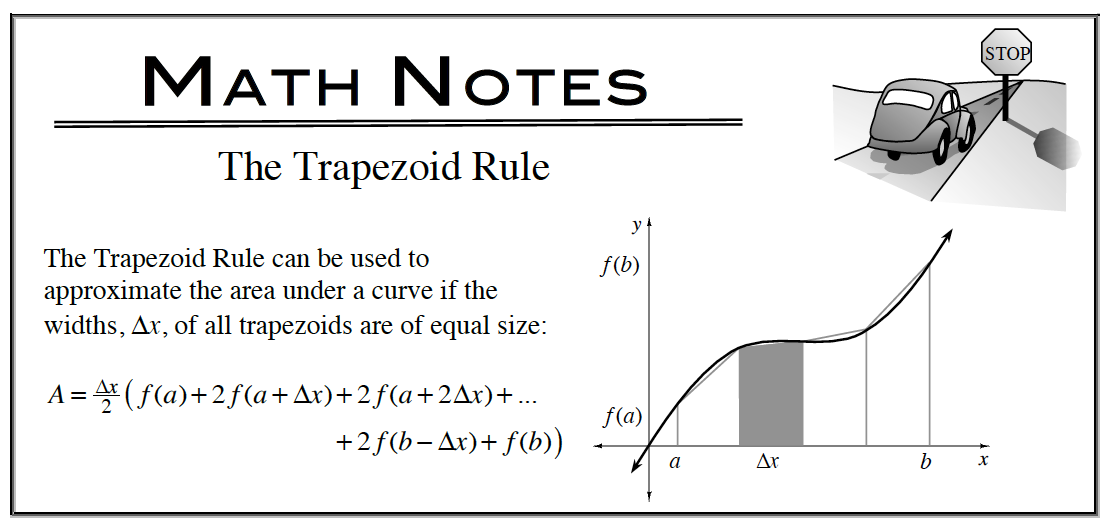### Home > CALC3RD > Chapter Ch5 > Lesson 5.4.1 > Problem5-147

5-147.

A function $f$ is continuous over the interval $[2, 8]$ and has values that are given in the table at right. Homework Help ✎

1. Using the sub-intervals $[2, 5], [5, 7]$, and $[7, 8]$, what is the trapezoidal approximation of $\int _ { 2 } ^ { 8 } f ( x ) d x$?2. Over which sub-interval is the average slope a minimum?

 I. $[2, 5]$ II. $[5, 7]$ III. $[7, 8]$

Test all answer choices and choose the lowest value. Recall that average slope is akin to saying average rate of change (AROC).

 $x$ $f \left(x\right)$ $2$ $10$ $5$ $30$ $7$ $40$ $8$ $20$